Question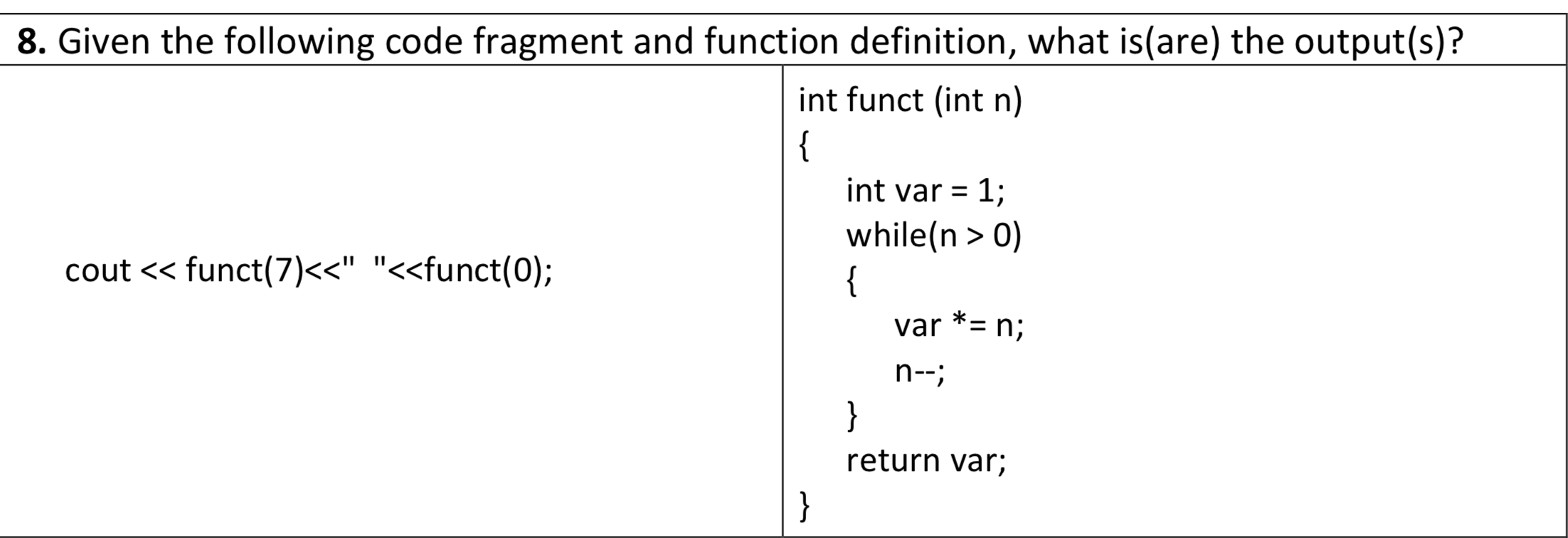// Screenshot of the code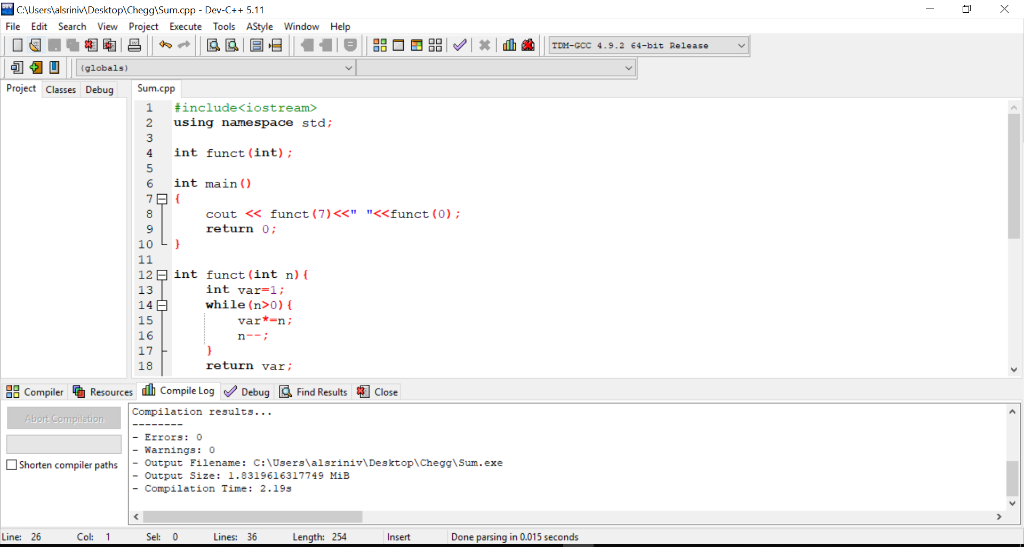// Sample output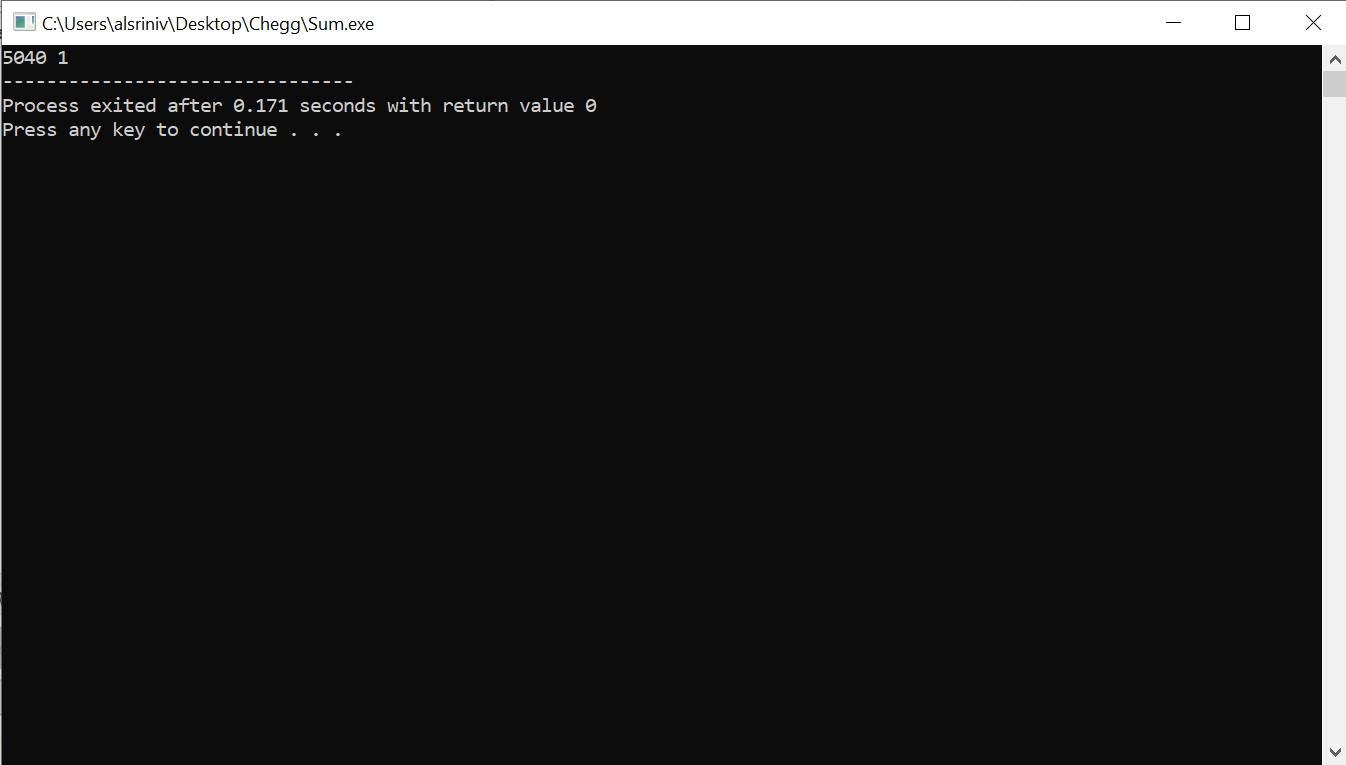// Code to copy

#include<iostream>
using namespace std;

int funct(int);

int main()
{
cout << funct(7)<<" "<<funct(0);
return 0;
}

int funct(int n){
int var=1;
while(n>0){
var*=n;
n--;
}
return var;
}

Explanation:

if n=7 the above code prints 7! value as 5040.

7!=7*6*5*4*3*2*1

#### Earn Coins

Coins can be redeemed for fabulous gifts.

Similar Homework Help Questions
• ### C++ Program What is the output of the following code fragment?(beta is of type int.) beta...

C++ Program What is the output of the following code fragment?(beta is of type int.) beta = 5; do { switch (beta) { case 1: cout <<'R'; break; case 2: cout case 4: cout << 'O'; break; case 5: cout << 'L'; } beta--; }while (beta>1); cout <<'X';

• ### A. What is the output of the following C++ code fragment? (all variables are of type...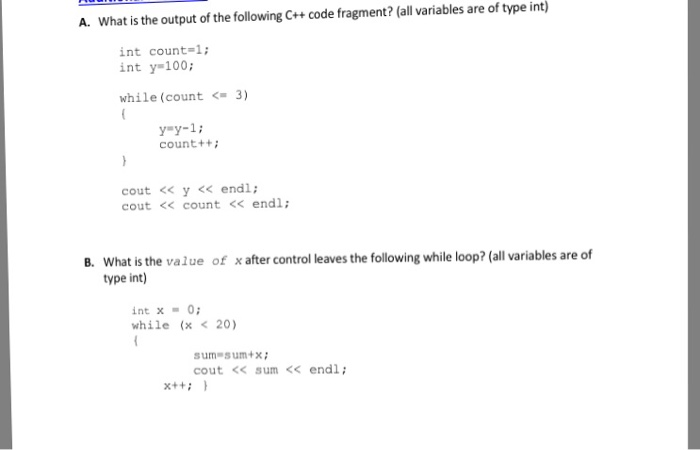A. What is the output of the following C++ code fragment? (all variables are of type int) int count-1; int y-100; while (count 3) y=y-1 ; count+t cout << y << endl; cout<< count <<endl What is the value of x after control leaves the following while loop? (all variables are of type int) B. int x0 while (x < 20) sum- sum+x cout << sum<< endl;

• ### 10) What is the output when the following code fragment is executed? void exam(int i) {cout...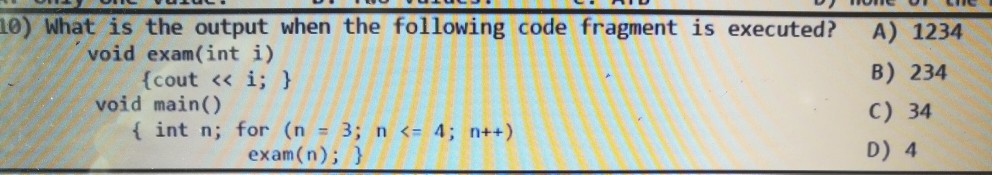10) What is the output when the following code fragment is executed? void exam(int i) {cout « i; } void main() { int n; for (n = 3; n <= 4; n++) exam(n); } A) 1234 B) 234 C) 34 D) 4

• ### What is the output of the following code fragment? int i = 1; while( i <=...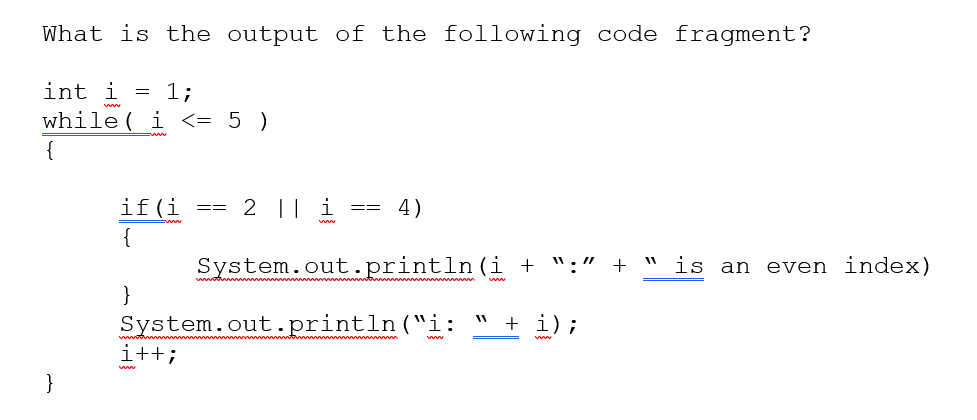What is the output of the following code fragment? int i = 1; while( i <= 5 ) if(i == 2 || i == 4) System.out.println(i + ":" + " is an even index) System.out.println("i: " + i); i++;

• ### Given the function definition, which of the following are correct? int func(int n, double d) {...

Given the function definition, which of the following are correct? int func(int n, double d) { int j = n; double sum = 0; while( j >= 0) { sum += d; -j; } return sum; } It compiles but computes none of these returns 7+2 returns 7! returns 7*2

• ### 12) 8 pts. Find the errors in the following code fragment and correct them. #i nclude...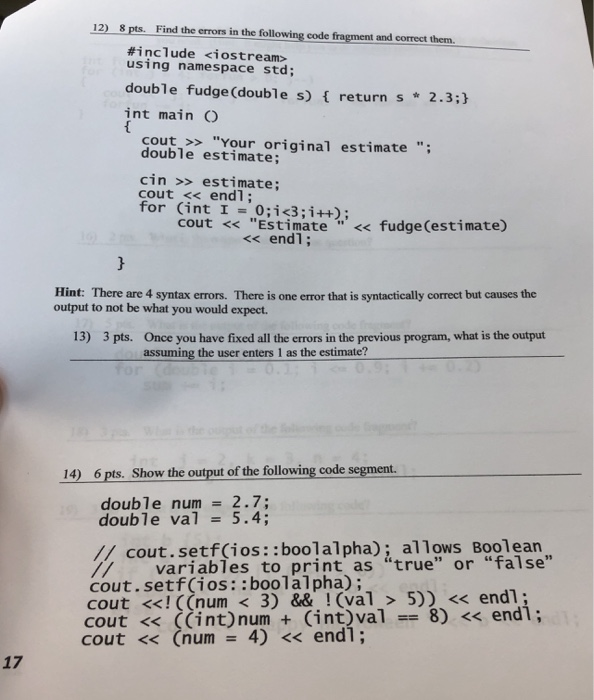12) 8 pts. Find the errors in the following code fragment and correct them. #i nclude <iostream> using namespace std; double fudge (double s) f return s 2.3; int mainO cout >> "Your original estimate" double estimate; cin >> estimate; cout << endl; for (int 1 = 0;1c3;i++); cout << "EStimate' < fudge(estimate) <<endl Hint: There are 4 syntax errors. There is one error that is syntactically correct but causes the output to not be what you would expect. Once...

• ### I don’t understand can you help 5. Given the definition and code fragment: (2 points int...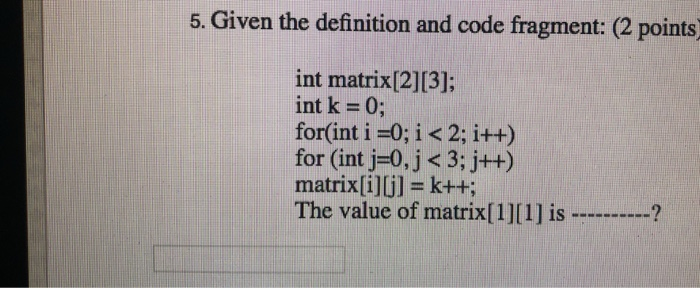I don’t understand can you help 5. Given the definition and code fragment: (2 points int matrix13]; int k = 0; for(int i =0; i < 2; i++) for (int j=0, j < 3 ) matrix[i]lj]-k++; The value of matrix is

• ### What is the output from each of the following segments of C++ code? Record the output...

What is the output from each of the following segments of C++ code? Record the output after the “Answer” prompt at the end of each program fragment. Assume all variables have been suitably declared. (Each problem is worth 3 points.) 1. for (int j = 25; j > 16; j -= 3)        cout << setw(5) << j;     Answer: 2. int sum = 0;       for (int k = -2; k <= 2; k++)         sum = sum +...

• ### QUESTION 8 What (if anything) will be the output of the following code: int count =...

QUESTION 8 What (if anything) will be the output of the following code: int count = 3; while (count++ <= 6) { System.out.print(++count + " "); } 3 4 5 6 4 5 6 7 3 4 5 6 7 5 7 4 6 The above code contains a syntax error and will not run. 8 points    QUESTION 9 What (if anything) will be the output of the following code: int count = 0; while (count < 5) {...

• ### Please use induction Consider the following code fragment: int i -1; int s=1; while (i <-...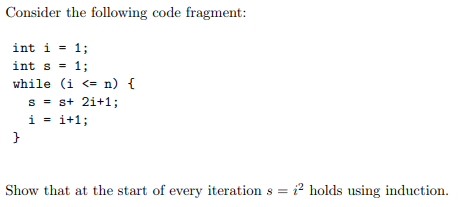Please use induction Consider the following code fragment: int i -1; int s=1; while (i <- n) Show that at the start of every iteration holds using induction